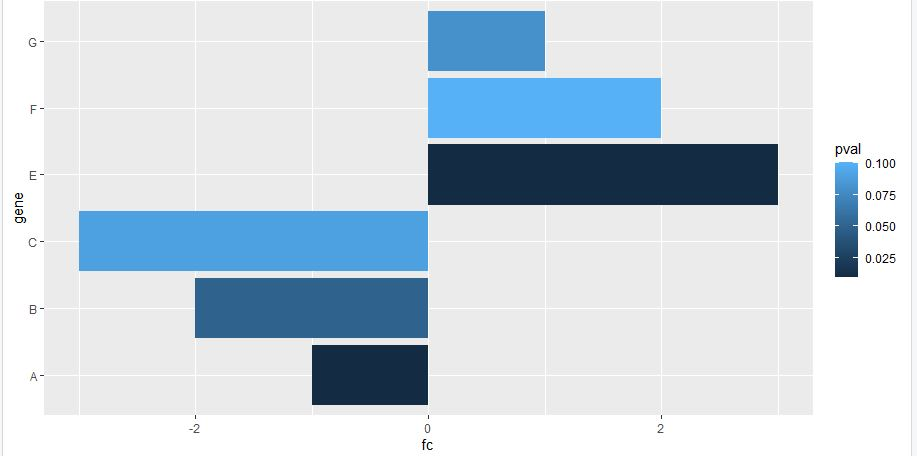Barplot in R for differential expression analysis
1
0
Entering edit mode
9 months ago

Hi everyone and happy holidays, I wondered if there's a way of graphical representing DE analysis in terms of logFC, in particular I've a list of differential expressed miRNAs, each of them associated to a specific logFC and P-values. How can I use thebarplot function to associate a bar for each miRNA, having the length of the bar proportional to the corresponding logFC value and the color with p-value? I would like to have a result similar to this, something I can easily get with an enrichment analysis. Do you recommend representations? It is important to me to compare different logFC values through the miRNAs. Thank you very much.

R rna-seq differential expression graph • 1.1k views
1
Entering edit mode

1
Entering edit mode
9 months ago
Hamid Ghaedi ★ 1.7k

This would create what you asked for, BUT for gene expression visualization bar plot is NOT a smart way to do so. Please follow @ATpoint comment (volcano plot) for visualization. Here is sample code for making volcano plot using ggplot package.

df <- data.frame(gene = c("A", "B", "C","E", "F", "G"), fc = c(-1,-2,-3,3,2,1), pval = c(0.01, 0.05, 0.09, 0.01,0.1,0.08))
ggplot(data=df, aes(x=gene, y=fc, fill = pval)) +
geom_bar(stat="identity") +
coord_flip()0
Entering edit mode

Really helpful, thank you very much for the support